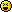# ForumCS2DScriptsPig script

#Pig script

9 replies
Goto Page1kalis
User
Offlinehello every one
Code:
1
2
3
4
5
6
7
8
9
10
11
12
13
14
15
16
17
18
check_x = math.floor(pig*32+16)
check_y = math.floor(pig*32+16)

if check_x < map('xsize')*32 and check_y < map('ysize')*32 then
if tile(check_x/32,check_y/32,'walkable') then
pig = check_x
pig = check_y
rot_change = 0
else

pig = fix_x
pig = fix_y
rot_change = 2
end

end

imagepos(pig.img,pig,pig,autorot)

why pig image doesn't appear ?
i think the fail in "math.floor" !
And how i can make my pig random move on the ground ?
mafia_man
User
OfflineCould you post whole script, because we don't know which value represents what. For example pig is it a tile sized value or pixels?
kalis
User
Offlineok here
Code:
1
2
3
4
pig = { pigx, pigy}

pig = (map('xsize')*32) / 2
pig = (map('ysize')*32) / 2
Infinite Rain
Reviewer
OfflineI think it's better for you to show us the full script.
A thousand may fall at your side, ten thousand at your right hand, but it will not come near you. You will only look with your eyes and see the recompense of the wicked. - Psalm 91:7-8 ESV
mafia_man
User
OfflineYour code is totally brainfuck I don't understand what's it going to do.

Code:
1
2
3
4
5
6
7
8
9
10
11
12
13
14
15
check_x = math.floor(pig / 32);
check_y = math.floor(pig / 32);

if check_x < map("xsize") and check_y < map("ysize") then
if tile(check_x, check_y, "walkable") then
pig = check_x * 32 + 16;
pig = check_y * 32 + 16;
rot_change = 0;
else
pig = fix_x;
pig = fix_y;
end
end

imagepos(pig.img, pig, pig, autorot);
EngiN33R
Moderator
OfflineWhy do you perform the tile to pixel conversion on pixel coordinates? According to your second post, the position is the middle of the map in pixels, yet you multiply that position by 32 and add 16 as if it were in tiles. Again, as said earlier, without the full code we aren't much help.
I code, therefore I exist. | Visit my blog for Lua tips and other interesting info
kalis
User
Offlineok full script
Code:
1
2
3
4
5
6
7
8
9
10
11
12
13
14
15
16
17
18
19
20
21
22
23
24
25
26
27
28
29
30
31
32
33
34
35
36
37
38
39
40
41
42
43
44
45
46
47
48
49
50
51
52
53
54
55
56
57
58
59
60
61
62
63
64
65
66
67
68
pig = { pigx, pigy, pigeat, pigwater, pighap}

pig = (map('xsize')*32) / 2
pig = (map('ysize')*32) / 2
pig.img = 0
local rot_change = 0
pig.rot = 45
pig.speed = 1
----change image you want !--------

local fix_x,fix_y,npc_x,npc_y

function restart_value(mode)
if pig.img ~= 0 then
freeimage(pig.img)
end

pic.img = 0
pig = (map('xsize')*32) / 2
pig = (map('ysize')*32) / 2
end

local autorot

function move()
if pig.img == 0 then

imagealpha(pig.img,1)

end
if pig.img ~= 0 then

local angel = math.deg(rot)
autorot = math.random(-2,2)*math.pi/2
if rot_change > 0 then
pig.rot = math.random(1,180)
else
pig.rot = pig.rot
end
check_x = math.floor(pig*32+16)
check_y = math.floor(pig*32+16)
fix_x = pig - math.sin(rot) * pig.speed
fix_y = pig + math.cos(rot) * pig.speed/3
if check_x < map('xsize')*32 and check_y < map('ysize')*32 then
if tile(check_x/32,check_y/32,'walkable') then
pig = check_x
pig = check_y
rot_change = 0
else

pig = fix_x
pig = fix_y
rot_change = 2
end

end

imagepos(pig.img,pig,pig,autorot)

end
end
Me Gusta
User
OfflineTo enable this pig system to who mod?
kalis
User
Offlinewhat you mean ?
Assasin ezio
User
Offlineno1﻿